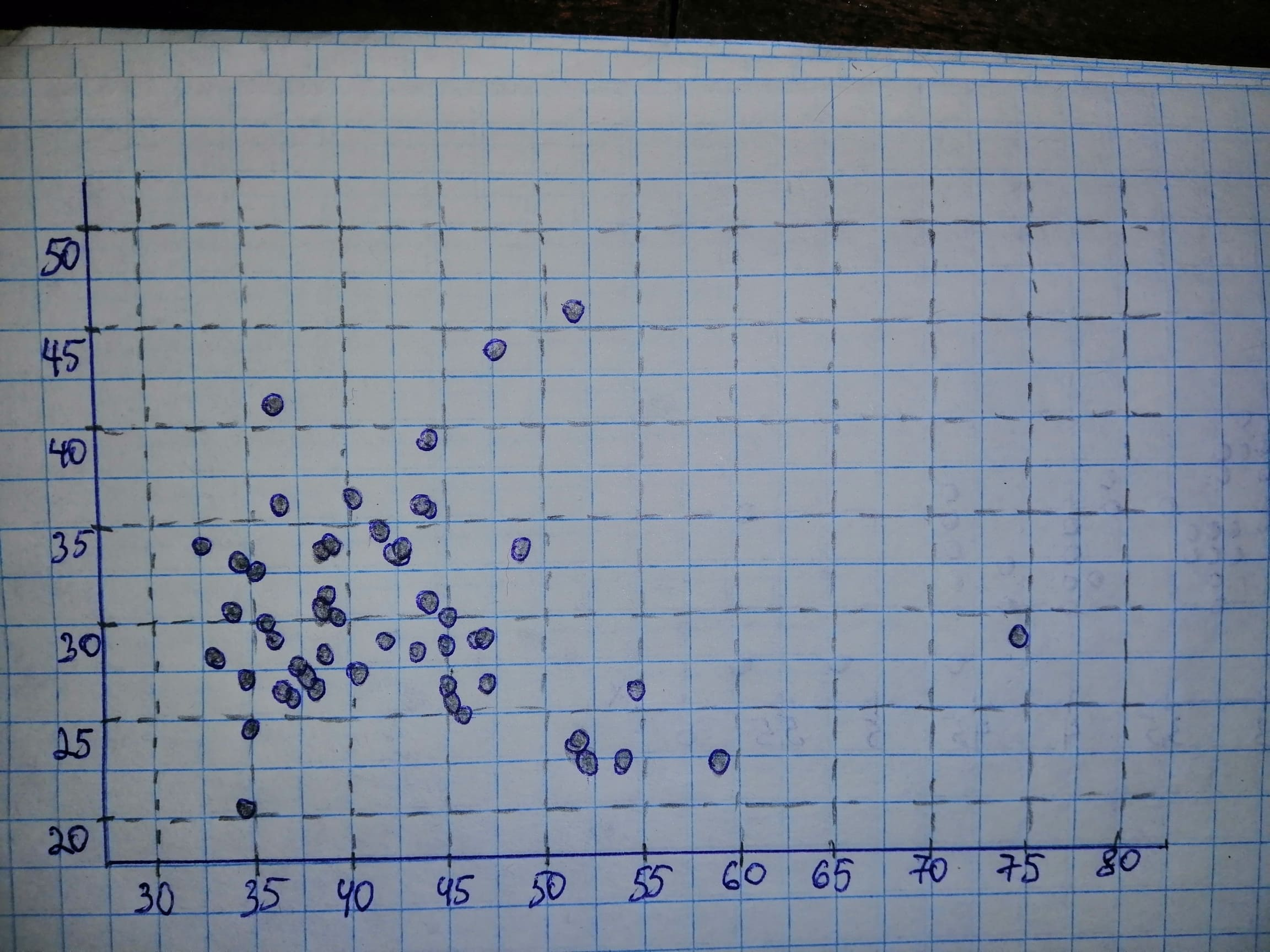Question# Does a higher state per capita income equate to a higher per capita beer consumption? From the document Survey of Current Business, published by the U

Scatterplots
ANSWEREDGiven: $$n=\ \text{Sample size}\ =51$$ a) Income is on the horizontal axis and Beer is on the vertical axis.b) It is reasonable to find a regression line for the data if there is no strong curvature present in the scatterplot. We note that there is strong curvature in the scatterplot of part (a) and thus it is not reasonable to find a regression line for the data. c) Not applicable, because it is not reasonable to find a regression line by part (b). d) Not applicable, because it is not reasonable to find a regression line by part (b). e) Not applicable, because it is not reasonable to find a regression line by part (b). f) Not applicable, because it is not reasonable to find a regression line by part (b).